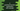# putchar() method explanation with example## putchar method in C explanation with example:

The putchar method in C is used to write a character to stdout. In this post, we will learn how to use this method with examples.

### Definition of putchar:

The putchar method is defined as like below:

``int putchar(int c)``

Here, c is the character to write. It converts the character to unsigned char before it is written.

It is defined in stdio.h. So, we have to include this header file to use putchar.

### Return value of putchar:

It returns the character written on success. On failure, it returns EOF.

### Example of putchar:

Let’s try putchar with an example:

``````#include <stdio.h>

int main()
{
for (char c = 'a'; c <= 'z'; c++)
{
putchar(c);
}
}``````

In this example, we are using a for loop that iterates from c = ‘a’ to c = ‘z’ and inside the loop it prints the current value of c.

If you run this program, it will print:

``abcdefghijklmnopqrstuvwxyz``

### Example of putchar with integer values:

We can also pass integer values to putchar. Let’s take a look at the below program:

``````#include <stdio.h>

int main()
{
for (int i = 65; i < 91; i++)
{
putchar(i);
}
}``````

It will convert the integer values to unsigned character. So, this program will print:

``ABCDEFGHIJKLMNOPQRSTUVWXYZ``

### Can we use putchar in C++ program:

We can use putchar method in C++ programs as well. We have to use cstdio.

``#include <cstdio>``